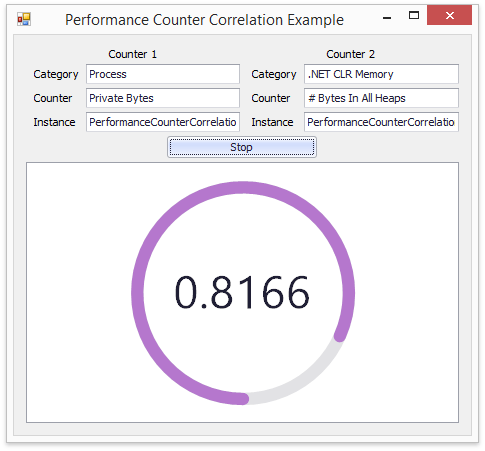.NET Framework 4.5.2+

# How to: Use Worksheet Data Bindings to Log and Process Data

This example illustrates the use of the Workbook instance to perform calculations on data bound to an external data source. The calculation result is located in the cell exposed as the data source for the GaugeControl control.

The application collects data from performance counters, logs them into a worksheet, calculates the correlation coefficient between data sets and gives a visual display of the coefficient value.

The source data are pairs of System.Diagnostics.PerformanceCounter object values. Performance samples (TwoCounterSample objects) are collected at the time interval specified by the timer. The samples are stored in the collection. A WorksheetProcessor object instance is created to process the collection of samples. A WoksheetProcessor includes a Workbook with a Worksheet containing a table bound to the performance data collection. The TableCollection.Add method is used to create a table bound to data.

The CORREL statistical function calculates the correlation coefficient. The cell containing the calculation result is exposed as the data source using the CellRange.GetDataSource method.

The worksheet data source providing a correlation coefficient value is bound to the ArcScaleComponent.DataBindings and the LabelComponent.DataBindings collections of the circular gauge control. The gauge control shows the correlation dynamically.

The application window is shown at the following picture.``````public class WorksheetProcessor {
Workbook wb;

public WorksheetProcessor(object dataSource) {
wb = new Workbook();
wb.CreateNewDocument();
Worksheet sheet = wb.Worksheets;
sheet.Cells["E2"].Formula = "IFERROR(CORREL(Table1[Column1], Table1[Column2]),0)";
}

public object GetDataSource() {
return wb.Worksheets.Cells["E2"].GetDataSource();
}

public void SaveData(string fileNameBase) {
string fileName = String.Format("{0:yyyy-MM-ddTHH-mm-ss}{1}.xlsx", DateTime.Now, fileNameBase);
wb.SaveDocument(fileName, DocumentFormat.Xlsx);
}

}
``````